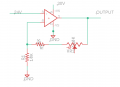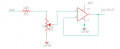# Voltage control with Ops Amp

#### Papabravo

Joined Feb 24, 2006
16,830
1. It needs more attention. Pins 3 & 5 are the power connections, and the difference must be at least as large as the range of the input signal.
2. Opamps start by computing the difference between the (+) or non-inverting input, and the (-) or inverting input.
3. This difference is then multiplied by a large number on the order of 10^5 or 10^6
4. In your configuration, the output at pin 4 will try to make the voltage at pin 2 equal to the voltage at pin 1 which is 24 volts. It may or may not be able to do this depending on things you have not shown on your schematic.
I think you need to go back to first principles and review the proper opamp configurations and how to analyze them. For example if you replace all three resistors and connect an appropriate power supply you will have a voltage follower and by definition pin 2 and pin 4 will both have the same voltage and the difference between pin 1 and pin 2 will be very close to zero.

#### Dave Newbie

Joined Jun 12, 2005
23
1. It needs more attention. Pins 3 & 5 are the power connections, and the difference must be at least as large as the range of the input signal.
2. Opamps start by computing the difference between the (+) or non-inverting input, and the (-) or inverting input.
3. This difference is then multiplied by a large number on the order of 10^5 or 10^6
4. In your configuration, the output at pin 4 will try to make the voltage at pin 2 equal to the voltage at pin 1 which is 24 volts. It may or may not be able to do this depending on things you have not shown on your schematic.
I think you need to go back to first principles and review the proper opamp configurations and how to analyze them. For example if you replace all three resistors and connect an appropriate power supply you will have a voltage follower and by definition pin 2 and pin 4 will both have the same voltage and the difference between pin 1 and pin 2 will be very close to zero.

Made some changes.#### crutschow

Joined Mar 14, 2008
27,755
That configuration will not allow an output voltage less then the 24V to to the plus input, it can only amplify the voltage.

One way to adjust the output from 0V to 24V is to tie the op amp output directly to the minus input to make it a follower, and then use the pot wiper to output 0V to 24V at the plus input.

How much op amp output current do you need?

•dcbingaman

#### Ramussons

Joined May 3, 2013
1,062
Hi, I am trying to control ops amp output voltage from 0 to 24 volt. Intent to use a digital Pot for VR1. Being a newbie at this like some advise.
what op amp to use to control 24V and if this schematic needs more attention.View attachment 244840
Why not use the Op Amp as a buffer and vary the + input voltage through a pot?

crutschow said the same•dcbingaman and BobaMosfet

#### Dave Newbie

Joined Jun 12, 2005
23
That configuration will not allow an output voltage less then the 24V to to the plus input, it can only amplify the voltage.

One way to adjust the output from 0V to 24V is to tie the op amp output directly to the minus input to make it a follower, and then use the pot wiper to output 0V to 24V at the plus input.

How much op amp output current do you need?
Looking at 40m

#### Dave Newbie

Joined Jun 12, 2005
23

#### Dave Newbie

Joined Jun 12, 2005
23

#### MrChips

Joined Oct 2, 2009
24,233
Connect the pot to 6V source and use an opamp with gain of 4.

•dcbingaman

#### Dave Newbie

Joined Jun 12, 2005
23
Connect the pot to 6V source and use an opamp with gain of 4.
Sorry for my ignorance. I understand to change from 24 to 6 volt. I did not understand the rest . If I am correct the feedback circuit remains the same.

#### LesJones

Joined Jan 8, 2017
3,498
As the "track" of your digital pot has a resistance of 10K if you connect one end of the track to ground and the other end of the track to +24 volts via a 30K resistor you will have 6 volts across the ends of the track. (You have creates a simple potential divider.)
You then need to configure the OP amp to multiply it's 0 to +6 volt input by 4 to create a 0 to +24 volts output.
Depending on the op amp you use you may need its negative supply pin to be a few volts negative of ground.
Note I have never used digital pots so I am assuming they behave like a normal pot except fot the voltage limitations.

Les.

#### Dave Newbie

Joined Jun 12, 2005
23
As the "track" of your digital pot has a resistance of 10K if you connect one end of the track to ground and the other end of the track to +24 volts via a 30K resistor you will have 6 volts across the ends of the track. (You have creates a simple potential divider.)
You then need to configure the OP amp to multiply it's 0 to +6 volt input by 4 to create a 0 to +24 volts output.
Depending on the op amp you use you may need its negative supply pin to be a few volts negative of ground.
Note I have never used digital pots so I am assuming they behave like a normal pot except fot the voltage limitations.

Les.

Is this correct#### ericgibbs

Joined Jan 29, 2010
13,654
hi,
Les states 30k.
E

#### LesJones

Joined Jan 8, 2017
3,498
Your op amp is only configured as a voltage follower so it's output will only be 0 to +6 volts. You need to read up on using op amps.
Edit. I have just noticed that Eric has spotted another error in your circuit that I had missed. As you have used a 10 K resistor instead of the 30 K that I suggested you will be applying 12 volts across the ends of the "track" on the digital pot. So it looks like you have to learn ohms law again.

Les.

Last edited:

#### Dave Newbie

Joined Jun 12, 2005
23

#### ericgibbs

Joined Jan 29, 2010
13,654
hi,
This is a LTS sim of the circuit, as you are learning, I have deleted the actual resistor values.

E

#### Attachments

•LesJones

#### Dave Newbie

Joined Jun 12, 2005
23

#### ericgibbs

Joined Jan 29, 2010
13,654
Can I confirm as 100 and 10 ohms for gain of 1.1
Hi Dave,
No, well off the mark, far too low a value and the wrong ratio.

Hint: what values did you use for the Resistor and potentiometer. ??

E

Non Inverting Gain equation: 1+ (Rfb/Rin)

Rfb= Feedback resistor, Rin = Input resistor

#### LesJones

Joined Jan 8, 2017
3,498
No.
An opp amp attempts to keep the voltage difference between the inverting and non inverting inputs to zero. You want the gain of the opp amp (With it's associated components.) to be x 4. R2 and R3 form a potential divider. You can't calculate the actual value of R2 and R3 but you can calculate the ratio of their values. So if we say that R2 is 10 K calculate the value of R3. I am not giving you the answer as I am trying to get you to understand op amp circuits. I you have to think about how it works you will be able to work out how to do the calculations for different configurations.

Les.

•Charles Tivendale

#### LesJones

Joined Jan 8, 2017
3,498